# NCERT Solutions for Class 7 Maths Chapter 2 Fractions and Decimals

### NCERT Solutions for Class 7 Maths Chapter 2 Fractions and Decimals Exercise 2.1 Ex 2.1 Class 7 Maths Question 1. Solve:Solution: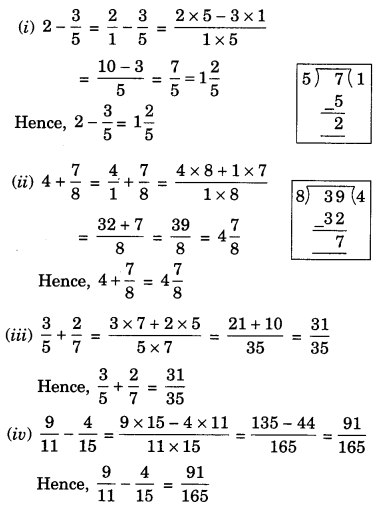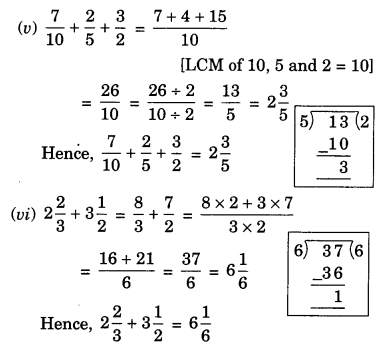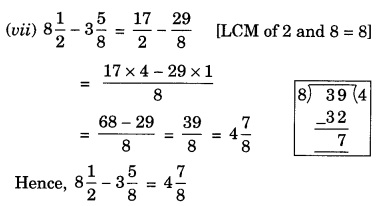Ex 2.1 Class 7 Maths Question 2.
Arrange the following in descending order:
$$(i) \frac{2}{9}, \frac{2}{3}, \frac{8}{21} \quad(i i) \frac{1}{5}, \frac{3}{7}, \frac{7}{10}$$
Solution: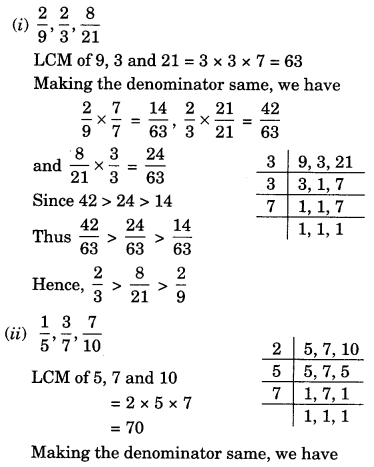Ex 2.1 Class 7 Maths Question 3.
In a “magic square” the sum of number in each row, in each column and along the diagonals is the same. Is this a magic square?Solution: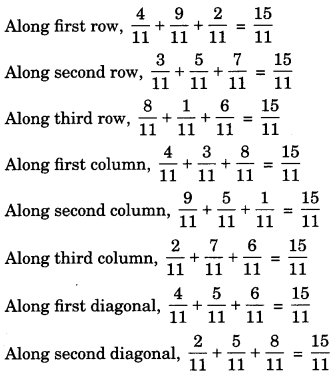Since, the sum of all the fraction row wise, column wise and the diagonal wise is same i.e. $$\frac{15}{11}$$.
Hence, it is a magic square.

Ex 2.1 Class 7 Maths Question 4.
A rectangular sheet of paper is $$12 \frac{1}{2}$$ cm long and $$10 \frac{2}{3}$$ cm wide. Find its perimeter.
Solution:Ex 2.1 Class 7 Maths Question 5.
Find the perimeter of (i) ∆ABE (ii) the rectangle BCDE in this figure. Whose perimeter is greater?
Solution:
(i) Perimeter of ∆ABE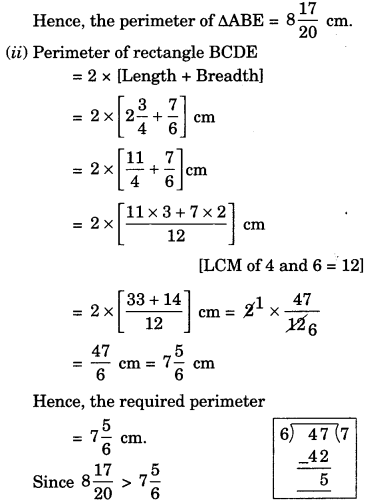Thus perimeter of ∆ABE is greater than the perimeter of the rectangle BCDE.

Ex 2.1 Class 7 Maths Question 6.
Salil wants to put a picture in a frame. The picture is $$7 \frac{3}{5}$$ cm wide. To fit in the frame, the picture cannot be more than $$7 \frac{3}{10}$$ cm wide. How much should the picture be trimmed?
Solution:
The width of the pictureHence, the required width to be trimmed $$=\frac{3}{10}$$ cm.

Ex 2.1 Class 7 Maths Question 7.
Ritu ate $$=\frac{3}{5}$$ part of an apple and the remaining apple was eaten by her brother Somu. How much part of the apple did Somu eat? Who had the larger share? By how much?
Solution:
Let the whole part of the apple be 1.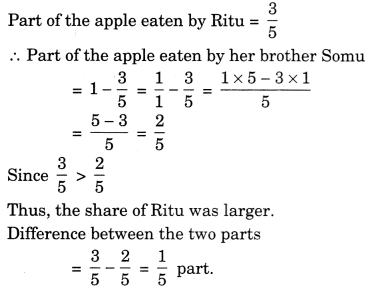Ex 2.1 Class 7 Maths Question 8.
Michael finished colouring a picture in $$\frac{7}{12}$$ hour. Vaibhav finished colouring the same picture in $$\frac{3}{4}$$ hour. Who worked longer? By what fraction was it longer?
Solution:
Time taken by Michael = $$\frac{7}{12}$$ hour
Time taken by Vaibhav = $$\frac{3}{4}$$ hour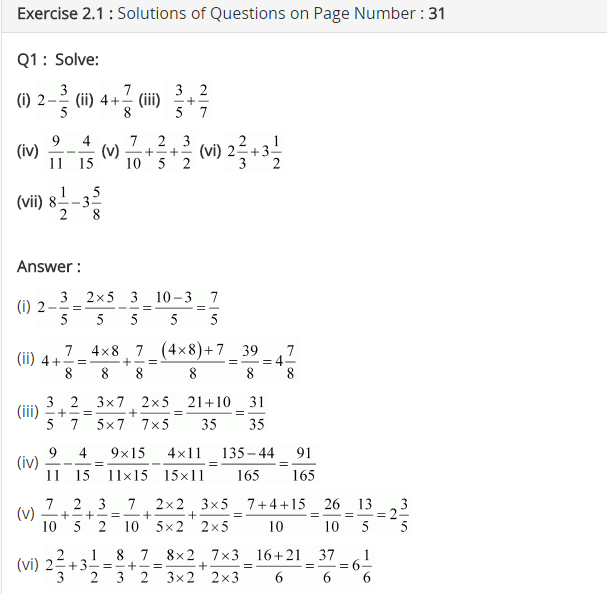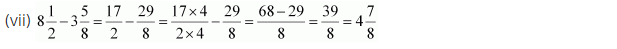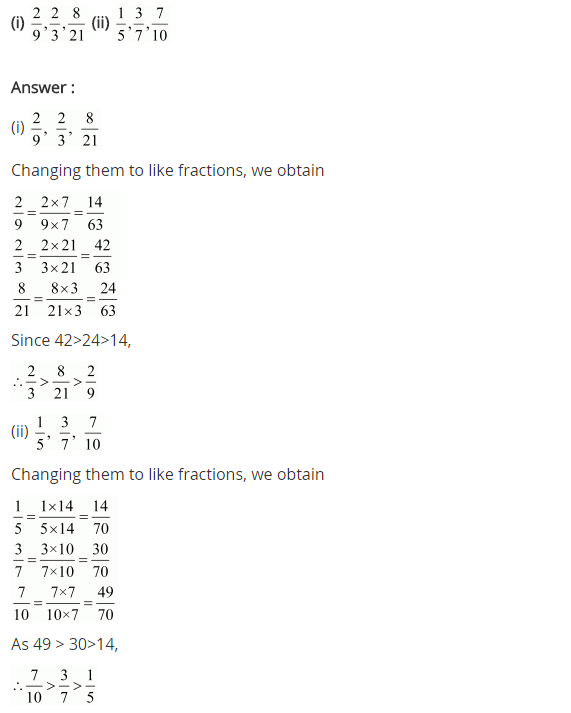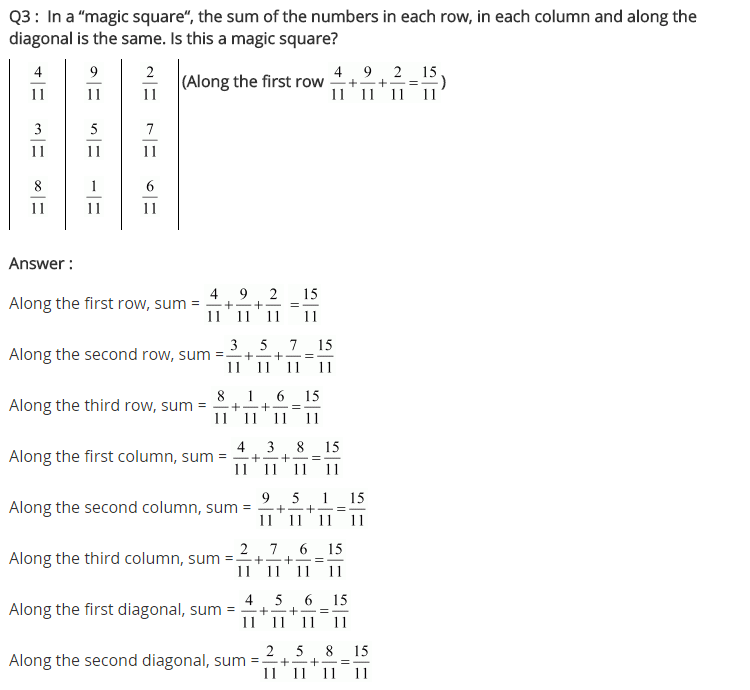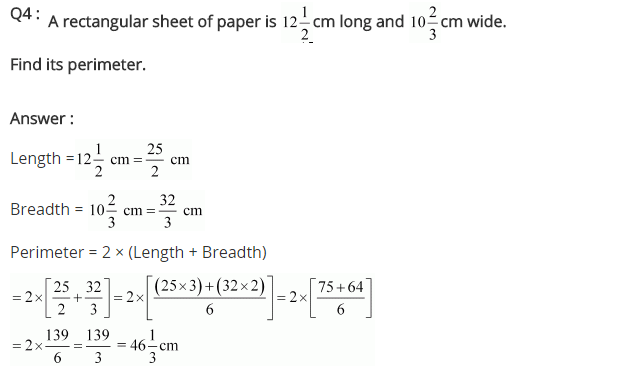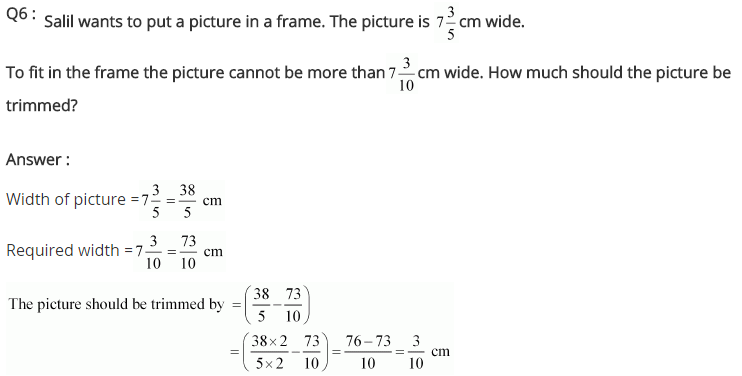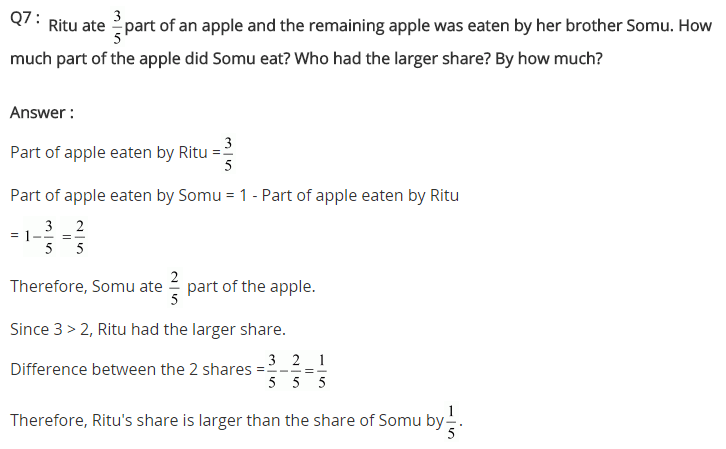+